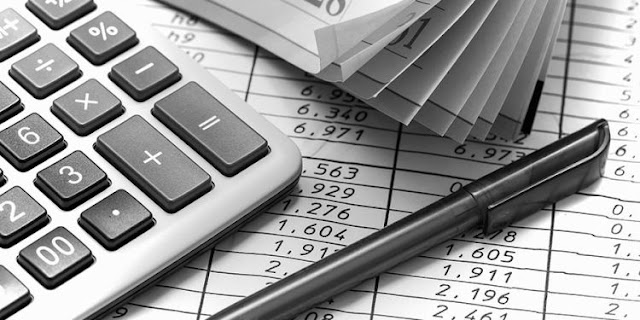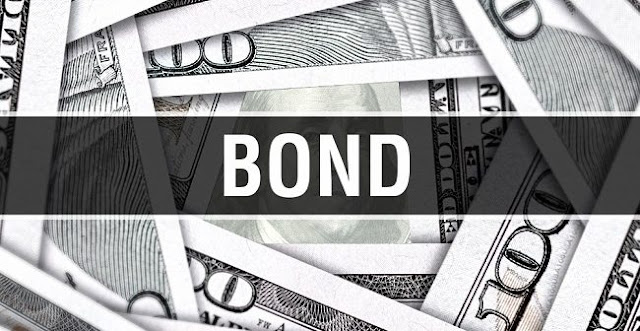# Bond Valuation: Understanding the Fair Value of Bonds for Informed InvestingBond Valuation: Understanding the Fair Value of Bonds for Informed Investing (photo: cloudinary.hbs.edu)

Bond valuation is the process of determining the fair value of a bond. This is an important concept for investors who are interested in buying or selling bonds, as it helps them to determine whether a particular bond is overpriced or underpriced. In this article, we will discuss the basics of bond valuation and how to calculate the value of a bond.

What is a Bond?photo: Veristrat

Before we dive into bond valuation, let's first define what a bond is. A bond is a debt security that is issued by a company, government, or other entity to raise capital. When you buy a bond, you are essentially lending money to the issuer for a specified period of time. In return for this loan, the issuer pays you interest on a regular basis and promises to repay the principal amount when the bond matures.

How Do Bonds Work?photo: businessterm.org

Bonds work by paying the investor a fixed rate of interest, known as the coupon rate, on a regular basis. This interest payment is typically made annually or semi-annually. When the bond reaches maturity, the issuer repays the principal amount to the investor.

The price of a bond can be affected by a variety of factors, including changes in interest rates, credit ratings of the issuer, and the time until the bond matures. As these factors change, the value of the bond can go up or down.

Read More: Credit Analysis for Bonds: An Essential Guide for Investors

Calculating Bond Valuationphoto: www.pbcom.com

The value of a bond is determined by discounting the future cash flows that the bond will generate back to their present value. The future cash flows include the periodic interest payments and the principal repayment at maturity.

The present value of the interest payments can be calculated using the following formula:

PV = C/(1+r)^1 + C/(1+r)^2 + C/(1+r)^3 + ... + C/(1+r)^n

Where: PV = Present Value C = Coupon Payment r = Discount Rate n = Number of Years

The present value of the principal repayment can be calculated using the following formula:

PV = F/(1+r)^n

Where: F = Face Value of the Bond n = Number of Years

Once you have calculated the present value of the interest payments and the principal repayment, you can add them together to determine the total value of the bond.

Example:

Let's say you are considering buying a bond that pays an annual coupon rate of 5% and has a face value of \$1,000. The bond has 10 years remaining until maturity and the current market interest rate is 4%.

To calculate the present value of the interest payments, we can use the formula:

PV = C/(1+r)^1 + C/(1+r)^2 + C/(1+r)^3 + ... + C/(1+r)^n

PV = \$50/(1+0.04)^1 + \$50/(1+0.04)^2 + \$50/(1+0.04)^3 + ... + \$50/(1+0.04)^10

PV = \$50/1.04 + \$50/1.0816 + \$50/1.1249 + ... + \$50/1.4802

PV = \$50.50 + \$46.39 + \$42.30 + ... + \$29.87

PV = \$446.10

To calculate the present value of the principal repayment, we can use the formula:

PV = F/(1+r)^n

PV = \$1,000/(1+0.04)^10

PV = \$1,000/1.4802

PV = \$675.56

The total value of the bond is the sum of the present value of the interest payments and the present value of the principal repayment:

Total Value = \$446.10 + \$675.

Read More: Understanding Yield Curve Bonds: A Guide to Investing in Bonds with Different Maturities

Factors Affecting Bond Valuationphoto: cdn.luatvietnam.vn

As mentioned earlier, the value of a bond can be affected by several factors. These factors include:

Interest Rates: The relationship between bond prices and interest rates is inverse. As interest rates rise, the value of existing bonds decreases. This is because new bonds that are issued at a higher interest rate offer better returns to investors than older bonds with lower interest rates.

Credit Ratings: The creditworthiness of the issuer also affects the value of a bond. Bonds issued by entities with a high credit rating are generally considered less risky and therefore command a higher price. Conversely, bonds issued by entities with a lower credit rating are considered riskier and will have a lower price.

Time to Maturity: The amount of time remaining until the bond matures can also affect its value. Generally, the longer the time to maturity, the higher the risk and the lower the value.

Call Features: Some bonds come with a call option that allows the issuer to redeem the bond before maturity. Bonds with a call option are generally considered riskier than bonds without a call option and may therefore have a lower value.

It is worth noting that while bond valuation is important, it is not the only factor to consider when investing in bonds. Investors should also consider the issuer's financial health, the purpose of the bond issuance, and any potential risks associated with the bond.

Additionally, it is important to note that bond valuation is not an exact science and there may be differences in opinion among investors about the fair value of a particular bond. This is why it is important to do thorough research and analysis before making any investment decisions.

Conclusion

Bond valuation is a key concept for investors to understand when investing in fixed-income securities. By calculating the fair value of a bond, investors can make informed investment decisions and potentially achieve better returns on their investment. However, it is important to consider all factors that may affect the value of a bond and to conduct thorough research before making any investment decisions.

Read More: Maximizing Your Investment Portfolio: The Benefits of Investing in Bonds During Low Interest Rates